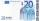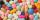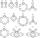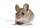# Basic functions - math word problems

1. StudyFor 7 hours study, she scored a 75 so if she made 93 how many hours would she need to study?
2. CacaoCacao contains 34% filling. How many grams of filling are in 130 g cacao.
3. Series and sequencesFind a fraction equivalent to the recurring decimal? 0.435643564356
4. Mother and daughterThe mother is four times older than her daughter. Five years ago, her daughter was seven times younger than her mother. How many years do they have now?
5. TreesA young tree is 16 inches tall. One year later, it is 20 inches tall. What is the percent increase in height?
6. Money splitAnton and Ferko got 2,500 euros together. The amount was split in 1:4 ratio. How much did each get?
7. Right triangle from axesA line segment has its ends on the coordinate axes and forms with them a triangle of area equal to 36 square units. The segment passes through the point ( 5,2). What is the slope of the line segment?
8. PillsIf it takes 20 minutes to run a batch of 100 pills how many minutes would it take to run a batch of 50 pills
9. Wagons and cranesSeveral of the same cranes unloaded 96 wagons. If there were 2 more cranes there would be less 8 wagons for each crane. How many cranes were here?
10. PumpsAfter the floods, four equally powerful pumps exhausted water from the flooded cellar in 6 hours. How many hours would take a drained out with three equally powerful pumps?
11. Magic numberThe number 135 split to two addends so that one addend was 30 greater than 2/5 the addend.
12. Big numberhat is the remainder when dividing number 10 to 47 - 111 by number 9?
13. Surface of cubesPeter molded a cuboid 2 cm, 4cm, 9cm of plasticine. Then the plasticine split into two parts in a ratio 1:8. From each part made a cube. In what ratio are the surfaces of these cubes?
14. 3 cats3 cats eat 3 mice in 3 days. How many mice will eat 10 cats in 10 days?
15. RatiosReduce the numbers: 50 in a 1:2 ratio 111 at a ratio of 2:3 70 at 10:50 560 at a ratio of 3:8
16. CuboidsTwo separate cuboids with different orientation in space. Determine the angle between them, knowing the direction cosine matrix for each separate cuboid. u1=(0.62955056, 0.094432584, 0.77119944) u2=(0.14484653, 0.9208101, 0.36211633)
17. Points on line segmentPoints P & Q belong to segment AB. If AB=a, AP = 2PQ = 2QB, find the distance: between point A and the midpoint of the segment QB.
18. RemainderA is an arbitrary integer that gives remainder 1 in the division with 6. B is an arbitrary integer that gives remainder 2 the division by. What makes remainder in division by 3 product of numbers A x B ?
19. Trapezoid - intersection of diagonalsIn the ABCD trapezoid is AB = 8 cm long, trapezium height 6 cm, and distance of diagonals intersection from AB is 4 cm. Calculate trapezoid area.
20. Family 8Father is 38 years old, daughter 12, son 14. How many years will father have as many years as his children together?

Do you have an interesting mathematical word problem that you can't solve it? Enter it, and we can try to solve it.

To this e-mail address, we will reply solution; solved examples are also published here. Please enter the e-mail correctly and check whether you don't have a full mailbox.

Please do not submit problems from current active competitions such as Mathematical Olympiad, correspondence seminars etc...